# A Simple Machine Learning Pipeline

This post contains the code that I used in my talk at Python Milano Meetup on June 22nd 2016. The talk was a quick overview of Pipeline, a nice API by scikitlearn to abstract your machine learning algorithm. It is based on the Boston Housing Data Set.

We'll just load the data set from sklearn.

``````from sklearn.datasets import load_boston
print housing_data.DESCR
``````

We might want to make it a Pandas dataframe to make things easier to handle.

``````import pandas as pd
df = pd.DataFrame(housing_data.data)
df.columns = housing_data.feature_names
df['PRICE'] = housing_data.target
``````The goal is to predict the PRICE variable given the other features. How does this variable distribute?

``````import matplotlib.pyplot as plt
df.PRICE.hist()
plt.xlabel('PRICE')
``````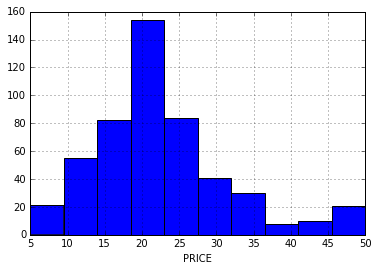{.alignnone .size-full .wp-image-74 width="378" height="271"}

Let's turn the dataframe into a ML-friendly notation.

``````X = df.drop('PRICE', axis=1)
y = df['PRICE']
``````

We will now define the metric that assess the accuracy of our algorithm/pipeline. Let's use the good old cross validation.

``````from sklearn import cross_validation
def evaluate_model(X, y, algorithm):
print 'Mean Squared Error'
scores = cross_validation.cross_val_score(algorithm, X, y,
scoring='mean_squared_error')
print -scores
print 'Accuracy: %0.2f' % -scores.mean()
``````

So, now, we can try a bunch of algorithms and see which one works best by calling evaluate_model. It is now time to implement a first algorithm. So, let's explore a bit the data set. Is there any pattern we can exploit?

``````plt.figure(figsize=(10,7))
plt.scatter(df['RM'], y)
plt.xlabel('Average number of rooms')
plt.ylabel('Housing price in \\$1000\'s')
plt.show()
``````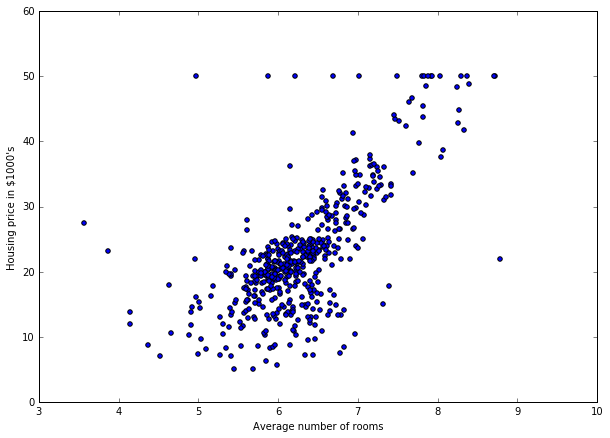{.alignnone .size-full .wp-image-78 width="610" height="438"}

As expected, there is a relation between the average number of rooms and the median price. So, let's build the first algorithm.

``````from sklearn.pipeline import make_pipeline
from sklearn.preprocessing import FunctionTransformer
from sklearn.linear_model import LinearRegression

def just_RM_column(X):
RM_col_index = 5
return X[:, [RM_col_index]]

pipe = make_pipeline(
FunctionTransformer(just_RM_column),
LinearRegression()
)
``````

How well does it perform?

``````evaluate_model(X, y, pipe)
'''Mean Squared Error [43.19492771 41.72813479 46.89293772] Accuracy:
43.94'''
``````

Can we visualize what the pipeline is actually doing?

``````def plot_model_RM(X, y, pipe):
X_train, X_test, y_train, y_test =
cross_validation.train_test_split(
X,
y,
test_size=0.33,
random_state=5
)
pipe.fit(X_train, y_train)
fake_X_train = np.array(X_train)
fake_X_train[:, 5] = np.linspace(min(fake_X_train[:, 5]),
max(fake_X_train[:, 5]), num=len(fake_X_train[:, 5]))
fake_X_test = np.array(X_test)
fake_X_test[:, 5] = np.linspace(min(fake_X_test[:, 5]),
max(fake_X_test[:, 5]), num=len(fake_X_test[:, 5]))
plt.figure(figsize=(20,7))
plt.subplot(1, 2, 1)
plt.scatter(X_train['RM'], y_train)
plt.scatter(fake_X_train[:, 5], pipe.predict(fake_X_train),
color='r')
plt.xlabel('Average number of rooms')
plt.ylabel('Housing price in \\$1000\'s')
plt.title('Train Data Set')
plt.subplot(1, 2, 2)
plt.scatter(X_test['RM'], y_test)
plt.scatter(fake_X_test[:, 5], pipe.predict(fake_X_test),
color='r')
plt.xlabel('Average number of rooms')
plt.ylabel('Housing price in \\$1000\'s')
plt.title('Test Data Set')
plt.show()

plot_model_RM(X, y, pipe)
``````{.alignnone .size-full .wp-image-84 width="1173" height="449"}

We now do a bit of feature engineering. We square the features.

``````def add_squared_col(X):
return np.hstack((X, X**2))

pipe = make_pipeline(
FunctionTransformer(just_RM_column),
LinearRegression()
)
``````

We evaluate this other pipeline.

``````evaluate_model(X, y, pipe)
'''
Mean Squared Error
[ 40.31207562 36.75642688 40.75444834]
Accuracy: 39.27'''
``````

And we see how the algorithm is fitting the data set.

``````plot_model_RM(X, y, pipe)
``````{.alignnone .size-full .wp-image-86 width="1165" height="449"} We now try a different model like a decision tree.

``````from sklearn.tree import DecisionTreeRegressor

pipe = make_pipeline(
FunctionTransformer(just_RM_column),
DecisionTreeRegressor(max_depth=3)
)
evaluate_model(X, y, pipe)
'''
Mean Squared Error
[ 57.28366371 61.5437311 84.32756118]
Accuracy: 67.72
'''
plot_model_RM(X, y, pipe)
``````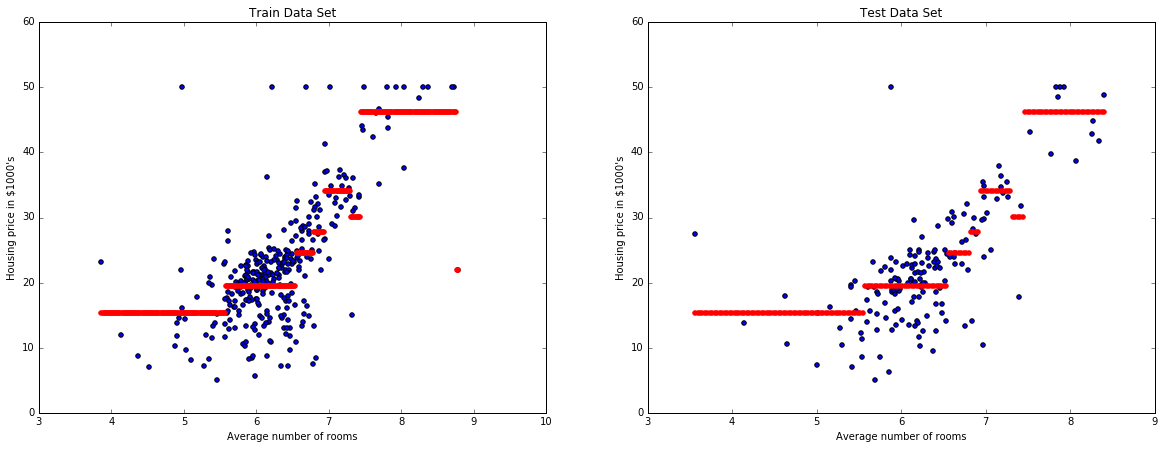{.alignnone .size-full .wp-image-87 width="1165" height="449"}

We now explore a second feature: INDUS.

``````plt.figure(figsize=(10,7))
plt.scatter(df['INDUS'], y)
plt.xlabel('Average number of rooms')
plt.ylabel('Housing price in \\$1000\'s')
plt.show()
``````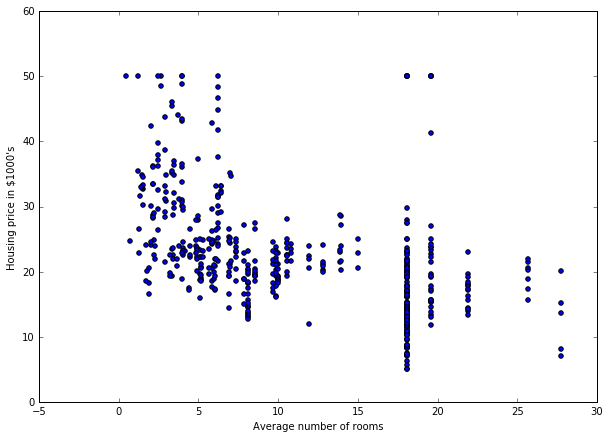{.alignnone .size-full .wp-image-89 width="610" height="438"}

So, we see another relation between INDUS and PRICE. So, let's add this second feature.

``````def RM_and_INDUS_cols(X):
RM_col_index = 5
INDUS_col_index = 2
return X[:, [RM_col_index, INDUS_col_index]]

pipe = make_pipeline(
FunctionTransformer(RM_and_INDUS_cols),
LinearRegression()
)

evaluate_model(X, y, pipe)
'''
Mean Squared Error
[ 32.3420789 31.4260901 35.95835866]
Accuracy: 33.24
'''
``````

Now, plotting a model in 3D needs a bit more effort.

``````def plot_model_RM_INDUS(X, y, pipe):
X_train, X_test, y_train, y_test =
cross_validation.train_test_split(
X,
y,
test_size=0.33,
random_state=5
)
pipe.fit(X_train, y_train)
X_test = np.array(X_test)
fig = plt.figure(figsize=(10,7))
ax = p3.Axes3D(fig)
x = X_test[:, 2]
y = X_test[:, 5]
z = y_test
ax.scatter(x, y, z, c='r', marker='o')
x = np.arange(min(x), max(x), (max(x) - min(x)) / 100.0)
y = np.arange(min(y), max(y), (max(y) - min(y)) / 100.0)
X, Y = np.meshgrid(x, y)
Z = np.zeros(X.shape)
fake_X = np.zeros((1, 10))
for i in range(X.shape):
for j in range(X.shape):
fake_X[0, 2] = X[i, j]
fake_X[0, 5] = Y[i, j]
Z[i, j] = pipe.predict(fake_X)
ax.plot_surface(X, Y, Z, alpha=0.2)
ax.set_xlabel('INDUS')
ax.set_ylabel('RM')
ax.set_zlabel('Price')

plot_model_RM_INDUS(X, y, pipe)
``````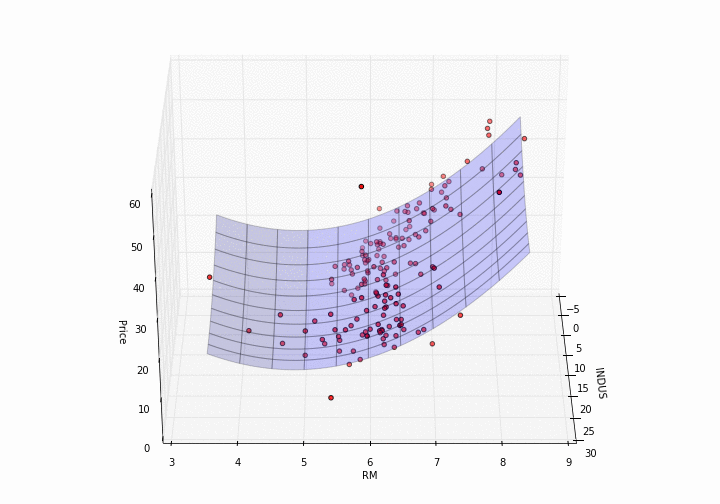{.alignnone .size-full .wp-image-91 width="720" height="504"}

How pretty is that?

The following step is to use all the features available. So, we move to a 13-dimensional feature vector.

``````pipe = make_pipeline(
LinearRegression()
)
evaluate_model(X, y, pipe)
'''
Mean Squared Error
[ 20.50009513 22.42870192 27.88911654]
Accuracy: 23.61'''
``````

The error got quite smaller. We cannot however plot the model in 13-dimensions. We will now re-use the function that adds a squared feature.

``````pipe = make_pipeline(
LinearRegression()
)
evaluate_model(X, y, pipe)
'''
Mean Squared Error
[ 16.7819682 14.599869 18.17785453]
Accuracy: 16.52'''
``````

Even better. Now, we will switch to a ridge-regressor (combined with a normalization of the features).

``````from sklearn.preprocessing import StandardScaler
from sklearn.linear_model import Ridge

pipe = make_pipeline(
StandardScaler(),